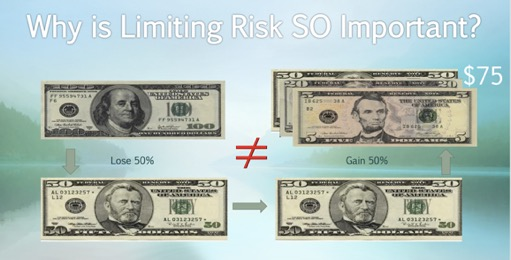Finance

# The mathematics of portfolio diversification

By

## The concept of diversification

Most people intuitively know the value of diversifying an investment or a portfolio.

“Do not put all of your eggs in one basket,” is a well-known saying.

However, the question becomes: “how many eggs and of which types do you put in each basket?”

• Portfolio diversification  requires precise quantitative tools.
• "Naïve diversification"  (randomly picking securities) already provides large benefits compared to holding only a handful of securities. Still, in order to properly allocate the components of a portfolio, one must resort to a more optimal quantitative optimization.

## Why is reducing risk so important?

A reality of investing is the fact that a negative return in one’s portfolio will hurt the long-term gains more than the equivalent positive return will help it grow. A typical example is the following: starting with a portfolio of \$100, if one experiences a 50% decrease and a 50% increase in the portfolio (the order in which this happens does not matter), the ending portfolio value will be \$75, in other words, a 25% loss! In order to recover from the initial 25% loss, a 100% gain would be needed.This is simply because for any return of magnitude “r,” the value of one dollar experiencing this return in both directions will always be   \$1(1+r)(1-r) = \$1(1-r^2) < \$1  and will thus result in a loss. Therefore, one must strive to minimize the risk level given the rate of return being targeted. Additionally, this asymmetry in the effect of negative and positive returns on the portfolio gets exacerbated with the magnitude of the fluctuations (r). As a practical numerical example in order to recover from a

• 25% loss, a 33% gain would be needed
• 50% loss, a 100% gain would be needed
• 60% loss, a 150% gain would be needed
• 95% loss, a 1900% gain would be needed

Essentially, the positive return needed to offset a negative one grows exponentially with the magnitude of the original decrease.

## What can be done about it?

Instead of randomly mixing securities (“naively” diversifying), one can take advantage of the securities’ statistics: their average returns, their volatility (risk) levels, and their respective correlations. Armed with this information and by varying the weights/allocations of each element in the portfolio, one can create a portfolio that will minimize the level of risk given the targeted portfolio return. This is often achieved using a computer program, but it can also be done using Excel, a tool that everyone has on their computer. Such an approach is referred to as a Markowitz portfolio optimization, in reference to Harry Markowitz and his Nobel Prize-winning paper on the topic. More bells and whistles can be added to the optimization, something described as “Modern Portfolio Theory.”

## The ultimate goal

The name of the game is to try to maximize the return while minimizing the risk or fluctuations in the portfolio. A convenient measure combining both concepts is something called the Sharpe Ratio, in reference to William Sharpe and his Nobel Prize-winning work on the concept. The Sharpe Ratio is also referred to as the “reward-to-risk ratio” because it consists of the return in the numerator and of the risk level (the standard deviation of the returns) in the denominator.

## In conclusion

While these tools are not by any means the only ones available to a portfolio manager and can also be used in combination with others (individual security picking using fundamental analysis, mutual funds or exchange-traded funds (ETFs) selection, momentum strategies, sector analysis, macroeconomic views, etc.), they do provide a powerful way to make one’s portfolio more efficient: experiencing less risk for the level of return being chased.